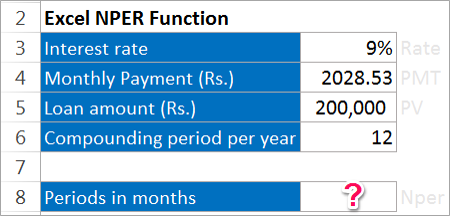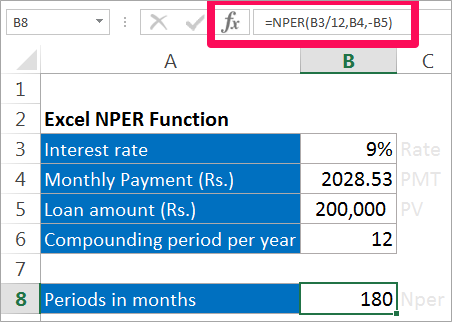# NPER function | Nper formulas in Excel in Hindi

## Nper function – Excel Formulas in Hindi

Nper function denotes the time period required to pay off the loans. This function will normally give answer to the question like suppose I have Rs. 200000 today how much time will it take double the money at interest rate of 6%. If we know the rate, Present value and the Annuity Payment we can easily find the number of years.

The formula for calculating Nper function

NPER(rate, pmt, pv, [fv], [type])

Where,

Nper stands for Number of years or the time period taken.

Rate stands for the interest rate at which is provided.

PMT stands for Payment Annuity.

PV stands for the Present Value.

FV stands for the Future Value.

Type stands for when the payment is made.

The arguments in the box brackets are optional.

### Example of Nper function

Rs 2028.53 is paid monthly for a loan of Rs 200,000. Interest rate is 9% and the payment needs to be done yearly. How much time will it take to pay off the loan.Solution:

Here, Rate = 9%

PMT= Rs 2028.53

PV = Rs 200,000

The other arguments are optional.